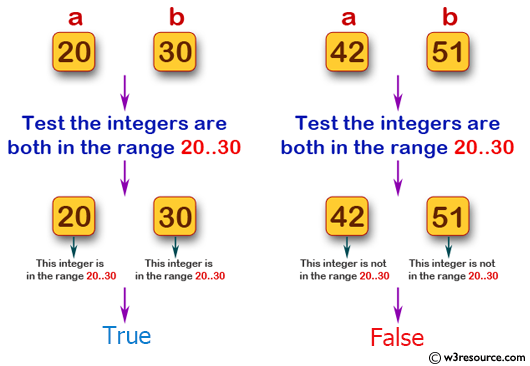﻿ Swift Basic Programming Exercise: Accept two integer values and test if they are both in the range 20..30 inclusive - w3resource# Swift Basic Programming Exercise: Accept two integer values and test if they are both in the range 20..30 inclusive

## Swift Basic Programming: Exercise-16 with Solution

Write a Swift program that accept two integer values and test if they are both in the range 20..30 inclusive, or they are both in the range 30..40 inclusive.

Pictorial Presentation:Sample Solution:

Swift Code:

``````func in3050(_ x: Int, _ y: Int) -> Bool {
if x >= 20 && x <= 30 && y >= 30 && y <= 40
{
return true
}
else if x >= 30 && x <= 40 && y >= 30 && y <= 40
{
return true
}
else
{
return false
}
}
print(in3050(20, 30))
print(in3050(30, 40))
print(in3050(42, 51))
```
```

Sample Output:

```true
true
false
```

Swift Programming Code Editor:

Improve this sample solution and post your code through Disqus

What is the difficulty level of this exercise?

﻿